astro automata focuses on automating astrophysics research with AI. It is both a personal research blog and collection of related content.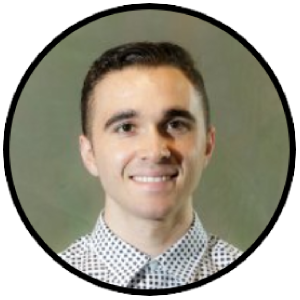Miles Cranmer

I’m a PhD candidate at Princeton trying to accelerate astrophysics with AI. Currently a research scientist intern at DeepMind.

New York City Email Twitter GitHub

Research (full list)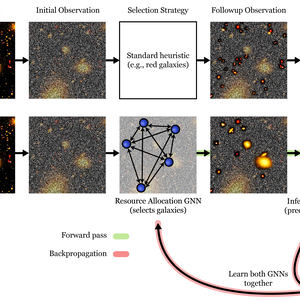Unsupervised Resource Allocation with GNNs

We show how to optimize resource allocation without knowing the true utility. We use this to learn a mock telescope observational survey from scratch.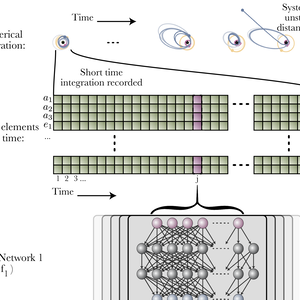Bayesian neural networks for planetary instability

We describe a Bayesian neural network architecture that can accurately learn to predict dynamical (chaotic) instability in compact planetary systems. The network demonstrates surprisingly robust generalization to 5-planet systems.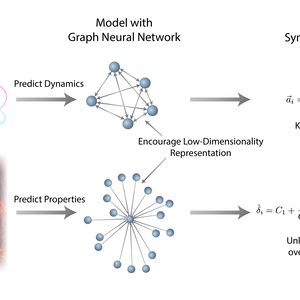Discovering Symbolic Models from Deep Learning

We describe a technique for converting a deep learning model into an analytic equation, focusing on graph networks. We validate it on injected force laws and Hamiltonians, and then discover a new equation to accurately predict the overdensity of dark matter from its environment.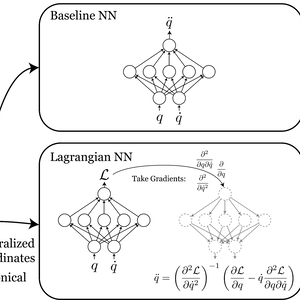Lagrangian Neural Nets

We show how one can learn a Lagrangian from data with a Neural Network. Such an architecture conserves energy in a learned simulator without requiring canonical coordinates.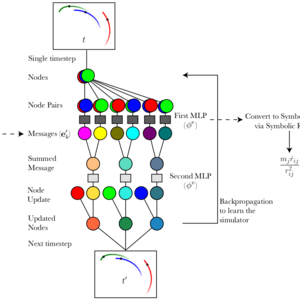Symbolic Models from Graph Networks

A graph network’s message-passing steps were motivated by Newtonian mechanics. But where is the physics actually hiding in the network? We explicitly show how one can extract learned forces from a graph net.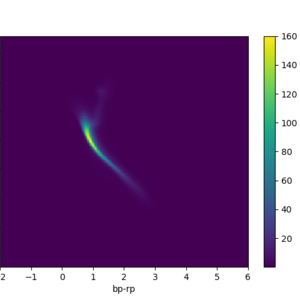Normalizing Flows for Stellar Isochrones

A normalizing flow is a highly flexible probability distribution, perfect for the nonlinear surface of an HR diagram. Here, we show how to learn a flow for Gaia’s HR diagram.

Posts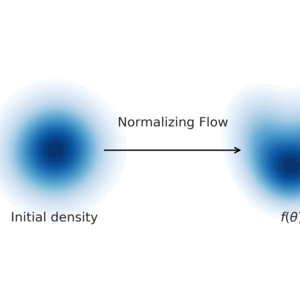An introduction to likelihood-free inference

I give an introduction to likelihood-free (simulation-based) inference for scientists.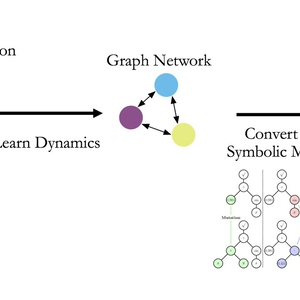The use and abuse of machine learning in astronomy

I argue that machine learning is both underused and overused in astrophysics.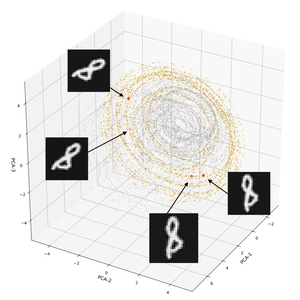Symmetries in Neural Networks

Related articles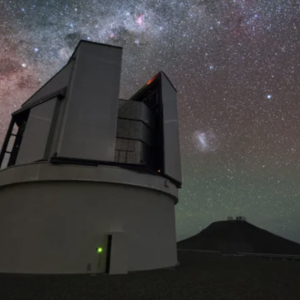How Self-Driving Telescopes Could Transform Astronomy

By Ryan F. Mandelbaum. What if an autonomously operating telescope, free from human biases and complications, could find the solutions we’ve been missing?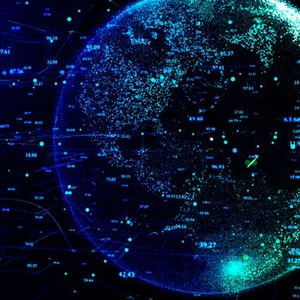AI Will Help Scientists Ask More Powerful Questions

By Pushmeet Kohli. Self-learning systems can discover hidden patterns in immense data sets, transcending what humans could ever find on their own